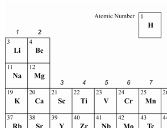# Science as as90944 v4 Demonstrate understanding of aspects of acids and bases Level 1, 4 Credits

 Date 23.04.2018 Size 37.62 Kb. #45982

 No Brain Too Small  SCIENCE 

SCIENCE AS AS90944 v4

Demonstrate understanding of aspects of acids and bases

Level 1, 4 Credits
This achievement standard involves demonstrating understanding of atomic structure, particle theory and rates of reaction relating to acids and base properties, uses and reactions.

Atomic structure

• periodic table; groups and periods

• periodic table; metals & non metals

• structure of the atom – particle location, charge and relative mass

• protons, neutrons & electrons

• electron arrangement of atoms of the first 20 elements (periodic table provided)

• relating the electron arrangement of atoms to their location in the periodic table

• comparing atoms in terms of p, n & e and mass

• ions

• definition

• electron arrangement of monatomic ions

• explanation of formation of ions (in terms of stability associated with a full valence shell)

• ionic bonding

• ionic compounds (a table of ions will be provided)

• names

• formulae

• explanation of formation of compounds (in terms of being electrically neutral)

Acids and bases

• acids

• HCl, H2SO4 & HNO3

• other acids may be included (names & formulae will be given in the question)

• release hydrogen ions in water

• pH of acids

• effects on indicators, including colour changes

• neutralisation; H+ + OH- → H2O

• reactions of acids with bases to form salts

• visual sign of reaction with carbonates; test for CO2 gas

• uses of acids

• bases

• metal oxides, hydroxides

• carbonates and hydrogen carbonates

• reactions of bases with acids to form salts

• difference between bases and alkalis

• pH of alkalis

• effects on indicators, including colour changes

• neutralisation

• carbon dioxide formation

• uses of bases

Rates of reaction and particle theory

• measurement of rate

• volume of gas produced

• loss of mass

• time for a colour to appear/disappear

• time for an X to be hidden by a reaction making a solid

• time for a given mass of solid to completely react

• collision theory

• explanation of rate in terms of

• effect of

• surface area

• concentration

• temperature

• catalysts

• explaining why reactions slow down & stop

• interpretation of graphs involving rates of reaction experiments

• Chemistry vocabulary

• symbols and conventions (including names and formulae)

• completing word equations

• completing given symbol equations

• writing balanced symbol equations

• graphical interpretations

• Table of ions

•  +1 +2 +3 –3 –2 –1 NH4+ Ca2+ Al3+ O2– OH– Na+ Mg2+ Fe3+ S2– Cl– K+ Cu2+ CO32– NO3– Ag+ Pb2+ SO42– HCO3– H+ Fe2+ Li+ Ba2+ Zn2+

• *Shaded ions – polyatomic ions

• Periodic Table

• Will show group numbers, atomic number and element symbol only – as shown in the sample below